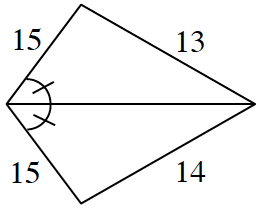### Home > CCG > Chapter 7 > Lesson 7.2.6 > Problem7-110

7-110.Carefully examine each diagram below and explain why the geometric figure cannot exist. Support your statements with reasons. If a line looks straight, assume that it is.

Review the triangle congruence conjectures.

1.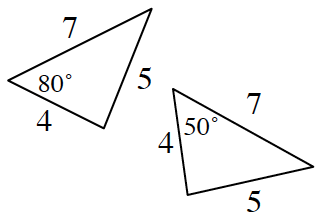1.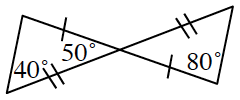1.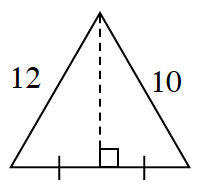• The triangles should be congruent by $\text{SSS}≅$, but $80°\ne50°$ and in congruent triangles all angles are equal as well.

• The triangles should be congruent by $\text{SAS}≅$, but $80°\ne90°$ and $40°\ne50°$ and in congruent triangles all angles are equal as well.

• The triangles should be congruent by $\text{SAS}≅$ , but $10\ne12$ and in congruent triangles all sides are congruent as well.

What do you know about isosceles triangles?

1.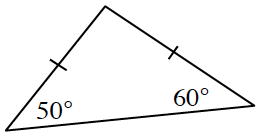1.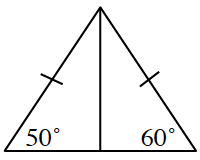1.